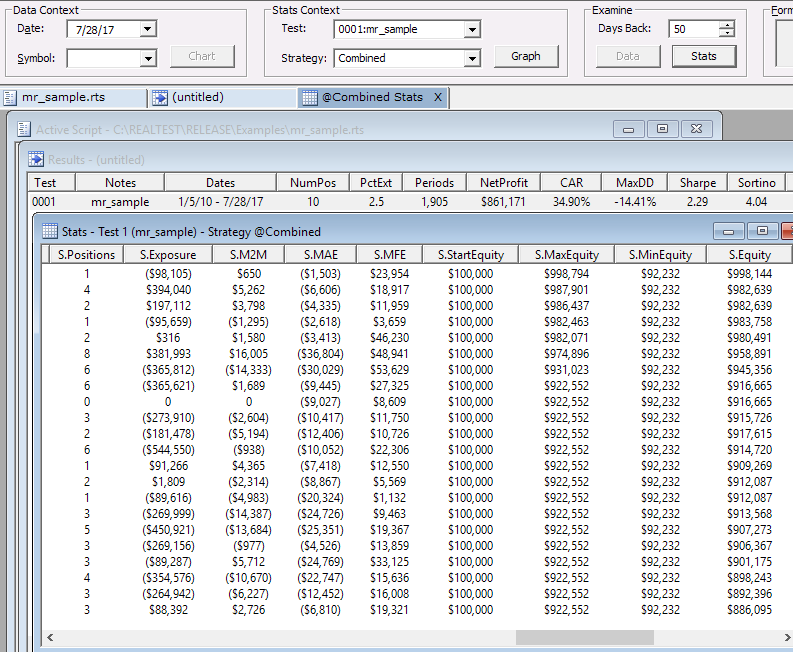Navigation: Realtest Script Language > Formula Syntax > Statistics Values in Formulas

RealTest provides a large set of daily test stat variables which can either be used directly or combined in expressions to calculate any conceivable system metric.

In order to give each of these stat items the most logical name without preventing that same name from being used as a column variable in Results or Graphs definitions, the built-in items all have names beginning with "S."

Stats variables are used in all of the formulas in RESULTS.RTS and GRAPHS.RTS - the default definitions for all Results and Graph windows.

Stats variables can also be used in any formula-based Strategy Element definition. When a test is being run, each day's daily stats variables are calculated and stored at the end of that day during the test.

This makes it possible, for example, for EntrySetup or ExitRule or Quantity formulas to include references to the strategy's current results so far.

A simple reference to a stat variable returns the value for the current day only. To obtain summary stats, use multi-bar functions with the stat variables.

For example, say a test is on day (bar) 100 and you want to know the overall profit factor so far. Profit factor is defined as dollars won / dollars lost. The RealTest syntax for dollars won is S.WinDlr and for dollars lost is S.LossDlr (both return positive values). The number of stat days so far in a test is available as S.Number. The profit-factor-so-far formula would therefore be Sum(S.WinDlr,S.Number) / Sum(S.LossDlr,S.Number). Or you might want to use a rolling 3-month profit factor, which would be Sum(S.WinDlr,63) / Sum(S.LossDlr,63).

The following stat properties are exceptions to the above rule and are accumulated internally, such that each day's value incorporates the entire test so far:

(It should be clear from the Min/Max prefixes that this is how these work.)

To make all of the above more concrete, do the following:

1.Run a test or open a results file

2.Open the debug panel

3.Select a test number and strategy name under "Stats Context"

4.Select a date that is within the range of dates for the test (and not a weekend or holiday)

5.Click "Stats" under "Examine"

The output will look something like the following image. There are more columns in the stats window than are visible here. Scroll right to see the rest of them.